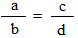Basic Math Formulas – Geometry, Probability, Arithmetic

0
243

Fractions formulas: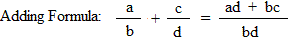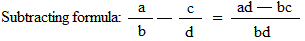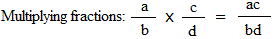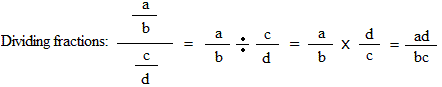Converting an improper fraction to a mixed number: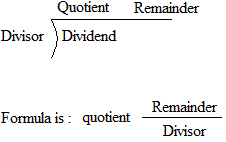Formula for a proportion: